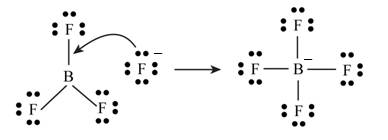# Define each of the following: a. Arrhenius acid b. Brønsted—Lowry acid c. Lewis acid Which of the definitions is most general? Write reactions to justify your answer.BuyFind

### Chemistry: An Atoms First Approach

2nd Edition
Steven S. Zumdahl + 1 other
Publisher: Cengage Learning
ISBN: 9781305079243BuyFind

### Chemistry: An Atoms First Approach

2nd Edition
Steven S. Zumdahl + 1 other
Publisher: Cengage Learning
ISBN: 9781305079243

#### Solutions

Chapter 13, Problem 1RQ
Textbook Problem

## Define each of the following:a. Arrhenius acidb. Brønsted—Lowry acidc. Lewis acidWhich of the definitions is most general? Write reactions to justify your answer.

Expert Solution

(a)

Interpretation Introduction

Interpretation: The definition of each term, Arrhenius acid, Bronsted-Lowry acid and Lewis acid is to be given. The most general definition from these terms is to be identified and the reactions are to be given for the justification of answers.

Concept introduction: An acid is a substance that turns litmus to red. It has a sour taste.

## Answer to Problem 1RQ

Answer

Arrhenius acid produces hydrogen ions in aqueous solution.

### Explanation of Solution

To define: Arrhenius acid.

The substance that produces hydrogen ions in aqueous solution is known as Arrhenius acid.

Arrhenius postulated the concept of acid. According to him “The substance that produces hydrogen ions in aqueous solution is known as acid.”

Expert Solution

(b)

Interpretation Introduction

Interpretation: The definition of each term, Arrhenius acid, Bronsted-Lowry acid and Lewis acid is to be given. The most general definition from these terms is to be identified and the reactions are to be given for the justification of answers.

Concept introduction: An acid is a substance that turns litmus to red. It has a sour taste.

## Answer to Problem 1RQ

Answer

Bronsted-Lowry acids are able to donate a proton.

### Explanation of Solution

To define: Bronsted-Lowry acid.

The substance that can donate a proton (H+) is known as Bronsted-Lowry acid.

Johannes Bronsted and Thomas Lowry proposed a model known as Bronsted-Lowry model. According to this model “The donor of proton (H+) is known as acid whereas acceptor of proton is known as base.”

Expert Solution

(c)

Interpretation Introduction

Interpretation: The definition of each term, Arrhenius acid, Bronsted-Lowry acid and Lewis acid is to be given. The most general definition from these terms is to be identified and the reactions are to be given for the justification of answers.

Concept introduction: An acid is a substance that turns litmus to red. It has a sour taste.

## Answer to Problem 1RQ

Answer

Lewis acids can accept a pair of electrons.

The most general definition of acid is given by Bronsted-Lowry model.

### Explanation of Solution

To define: Lewis acid.

The chemical species that accepts a pair of non-bonding electrons is known as Lewis acid.

The Gilbert Newton Lewis suggested acid-base theory. According to this theory “The species that can accept a pair of non bonding electrons is known as acid.”

The most general definition of acid is given by Bronsted-Lowry model because this model is applicable to the non-aqueous solution also.

Arrhenius acid,

The substance that produces hydrogen ions in aqueous solution is known as Arrhenius acid.

The dissociation reaction of HCl is,

HClH++Cl

The compound HCl is dissociated into H+ ion. Hence, it is an acid.

Bronsted-Lowry acid,

The donor of proton (H+) is known as acid.

The reaction of HCl with water is,

HCl(g)+H2O(l)H3O+(aq)+Cl(aq)

Lowry acid,

The chemical compound that accepts a pair of non-bonding electrons is known as Lewis acid.

The compound BF3 is electron deficient at central position,Figure 1

### Want to see more full solutions like this?

Subscribe now to access step-by-step solutions to millions of textbook problems written by subject matter experts!

### Want to see more full solutions like this?

Subscribe now to access step-by-step solutions to millions of textbook problems written by subject matter experts!© 2021 bartleby. All Rights Reserved.